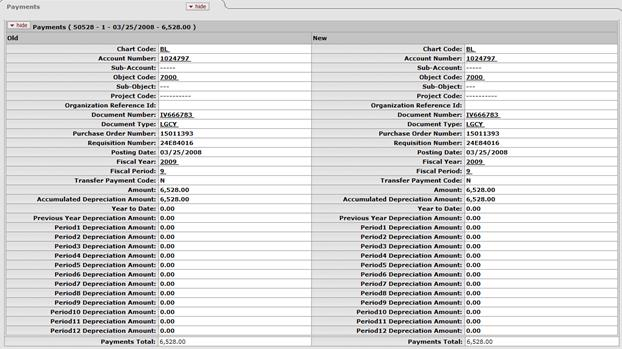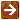﻿ Payments Tab
###### Payments TabThe information in this tab is retrieved from the database upon opening of the document and is view only.If more than ten payments exist for the asset, the user may view all payments via the Asset Payment Lookup. For more information about the lookup form, see Asset Payment Lookup.

Payments tab definition

 Description The chart code for the account that funded the asset or is the owner of the asset. Account Number The account number that paid for or owns the asset. Sub-Account An optional element of the accounting string that allows the user to track financial activity within a particular account at a finer level of detail. Object Code Used to classify the transaction and to identify asset categories such as movable, art and museum, buildings. In addition, the object code can be used to identify the type of funding, university, federal, or federally owned. Sub-Object An optional element of the accounting string related to the university object code that allows the user to create finer distinctions within a particular object code for a specific account. Project Code Used to identify particular transactions that might span multiple accounts. Organization Reference Id A reference identification number assigned by organization. Document Number The transactional document number that generated the capital expense. The type of document that generated the capital expense. Purchase Order Number Represents a written authorization for a vendor to ship goods or provide services based on conditions outlined in the purchase order (PO) document which becomes a legally binding contract once the vendor accepts it. Requisition Number The number of the document used to order the equipment. Posting Date The date the payment transaction was posted to the General Ledger. Fiscal Year The fiscal year the payment was posted to the General Ledger. Fiscal Period The fiscal period the payment was posted to the General Ledger. Transfer Payment Code This code is used to identify if the payment is eligible for depreciation. Amount The portion of the cost of the asset paid from the above account for the given payment. Accumulated Depreciation Amount The depreciation on the asset related to this payment. Year To Date The amount of depreciation for the current fiscal year. Previous Year Depreciation Amount The amount of depreciation for the prior fiscal year. Period 1 Depreciation Amount Depreciation amount for the 1st period (July) of the fiscal year. Period 2 Depreciation Amount Depreciation amount for the 2nd period (August) of the fiscal year. Period 3 Depreciation Amount Depreciation amount for the 3rd period (September) of the fiscal year. Period 4 Depreciation Amount Depreciation amount for the 4th period (October) of the fiscal year. Period 5 Depreciation Amount Depreciation amount for the 5th period (November) of the fiscal year. Period 6 Depreciation Amount Depreciation amount for the 6th period (December) of the fiscal year. Period 7 Depreciation Amount Depreciation amount for the 7th period (January) of the fiscal year. Period 8 Depreciation Amount Depreciation amount for the 8th period (February) of the fiscal year. Period 9 Depreciation Amount Depreciation amount for the 9th period (March) of the fiscal year. Period 10 Depreciation Amount Depreciation amount for the 10th period (April) of the fiscal year. Period 11 Depreciation Amount Depreciation amount for the 11th period (May) of the fiscal year. Period 12 Depreciation Amount Depreciation amount for the 12th period (June) of the fiscal year. Payments Total The total of payments made to date for this asset.

More: